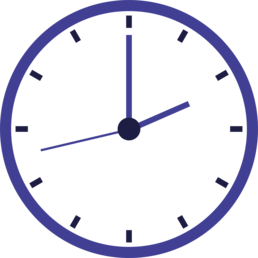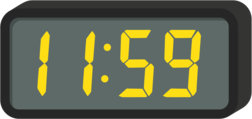meta name="description" content="Students practice telling time from analog and digitial clocks.">

# Telling Time Worksheets

How to Tell Time on an Analog Clock - An analog clock is the one that has moving hands to tell time. They typically have a round face with numbers 1 to 12 written on them. These clocks also feature two stick-like structures, which are commonly known as the hands. These hands move around the clock to show us time. Generally, analog clocks have three types of hands; the longest one tells us seconds and is known as the seconds hands, the comparatively shorter one tells us minutes and is known as the minute hands, and the shortest one tells us hours and is known as the hour hand.To tell hours, we have to look at the shortest hand. We notice the number at which the shortest hand is resting on, or which number it just passed. Note that all the hands move from a smaller number to a greater number. And when they reach 12, they start moving from 1. If your hour hand just passed 7, that means that we are in the 7th hour. For reading hours, we look at the large numbers on the clock. The minute hand is the one between the hour and second hands. When we have to read minutes, we do not look at the large numbers, but instead, we read the marks present in between the large numbers. We observe that which tick mark has the minute hour just passed if it has passed 1 tick mark, it will be read as 1 minute.

• ### Basic Lesson

Students learn how to identify analog time on a variety of clocks. Includes 3 practice problems.

• ### Independent Practice 1

Practice telling time and writing the numbers and words for time.

• ### Independent Practice 2

More practice on time in numbers to words. Also telling time in numbers and words. Match the time on the left with same time one the right.

• ### Homework Worksheet

10 problems sorted between telling time and rewriting time as words.

• ### Skill Quiz

Reviews all telling time skills in 10 problems. Includes a scoring key. Write the correct time below the clock.

• ### Homework and Quiz Answer Key

Answers for the homework and quiz.

Answers for the lesson and practice sheets.

• ### Basic Lesson

Students learn the significance of the minute and hour hands on clocks. Includes 2 practice problems. When using an analog clock (one with hands) remember that the short hand represents the hours and the long hand represents the minutes.

• ### Intermediate Lesson

Practice drawing the minute and hour hand on clocks.

• ### Independent Practice 1

Practice telling time and writing the numbers and words for time.

• ### Independent Practice 2

Students draw the minute and hour hands on clocks.

• ### Homework Worksheet

Walks students through both telling time and drawing hands on the clocks.

• ### Skill Quiz

6 problems ask students to tell time. 4 problems ask students to draw the hands on the clocks.

• ### Homework and Quiz Answer Key

Answers for the homework and quiz.

Answers for the lesson and practice sheets.

### How to Tell Time on a Digital Clock?Read the first number to determine the hour. In other words, the numbers before the colon represent the hour. Now, read the numbers on the right of the colon, which represents the minutes. Now, combine both of the numbers, and you should be able to tell the time.

#### Time and Math...

Did you know there is a great show on television about the relationship between time and math?
It's scheduled for prime time viewing at 2 o'clock, 3 o'clock, 5 o'clock, 7 o'clock and 11 o'clock.